Control Index

Laplace Transforms

Introduction

In control system design it is necessary, to analyse the performance and stability of a proposed system before it is built or implemented.   Many analysis techniques use transformed variables to facilitate mathematical treatment of the problem.   In the analysis of continuous time dynamical systems, this generally involves the use of Laplace Transforms

Applying Laplace Transforms is analogous to using logarithms to simplify certain types of mathematical operations.   By taking logarithms, numbers are transformed into powers of 10 or e (natural logarithms ).   As a result of the transformations, mathematical multiplications and divisions are replaced by additions and subtractions respectively.   Similarly, the application of Laplace Transforms to the analysis of systems which can be described by linear, ordinary time differential equations overcomes some of the complexities encountered in the time-domain solution of such equations.

Laplace Transforms are used to convert time domain relationships to a set of equations expressed in terms of the Laplace operator 's'.   Thereafter, the solution of the original problem is effected by simple algebraic manipulations in the 's' or Laplace domain rather than the time domain.

The Laplace Transform of a time variable f(t) is arrived at by multiplying f(t) by e -st and integrating from 0 to infinity..
f(t) must be a given function which is defined for all positive values of t.
s is a complex variable defined by... s = a +jω and j = sqrt (-1).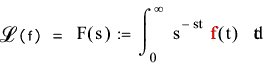A table of laplace transforms is available to transform real time domain variables to laplace transforms. The necessary operations are carried out and the laplace transforms obtained in terms of s are then inverted from the s domain to the time (t) domain.   This tranformation from the s to the t domain is called the inverse transform...

The contour integral which defines the inverse Laplace Transform is shown below... for reference only, for in practice, this integral is seldom used as table lookup are generally all the operations required for the inverse transform procedure..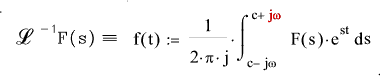Laplace Transform Operations.

 Operation f(t) F(s) Linearity x 1 f 1 (t) + x 2 f 2 (t) x 1 F 1 (s) + x 2 F 2 (s) Constant Multiplication a.f(t) a.F(s) Complex shift Theorem e ± a.t.f(t) F(sa.t ) Real shift Theorem f( t - T ) e -Ts F(s) for (T >= 0 ) Scaling Theorem f( t / a ) a F(as) First Derivative f' (t) sF(s)   -  f(0+) 2nd Derivative f'' (t) s 2 (F(s)   -  sf(0+)  -  f'(0+) 3rd Derivative f''' (t) s 3 (F(s)   -  s 2. f(0+)   -  s.f'(0+)  -  f''(0+) 4th 5th .. Derivative follow principles established above First Integral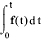(1/s).F.(s) Convolution Integral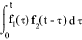F 1(s). F 2(s)

Table showing selection of Laplace Transforms.

 Laplace Transforms No Time Function = f(t) Laplace Transform = F(s) 1 d ( t )..Unit impulse 1 2 d(t - T)..Delayed impulse e -Ts 3 t ...Unit ramp 1 / s 2 4 t n n ! / s ( n+1 ) 5 e - at 1 / ( s + a ) 6 e at 1 / ( s - a ) 7 (1 / a) .(1 - e -at ) 1 / {s.( s + a )} 8 (t n / n !)e - at 1 /(s + a ) n - 1...n = 1,2,3,4,... 9 sin ωt ω / (s 2 + ω 2 ) 10 cos ωt s / (s 2 + ω 2 ) 11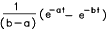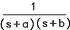12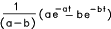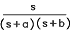13 (1 / ω 2) .(1 — cos ωt ) 1 / {s.( s 2 + ω 2 )} 14 (1 / a 2) .(a.t — 1 + e -at ) 1 / {s 2.( s + a )} 15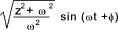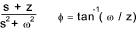16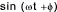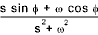17 u(t) or 1 ...Unit step 1/s 18 u(t - T)...Delayed step ( 1 / s )e -Ts 19 u(t) - u(t - T) or 1 ...Rectangular Pulse ( 1 / s ) (1- e -Ts ) 21 e — at cos ωt (s+a) / { (s+a) 2 + ω 2 } 22 (1 / a 2 )(1 — e -at — ate -at ) (1 / s) ( s + a) 2 23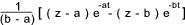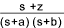24 1 / [( s + a ) 2 + ω 2 ] (1/ω).e -at sin ωt 25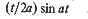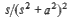26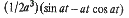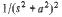Derivation of table values

examples on how the table values have been derived are provided below.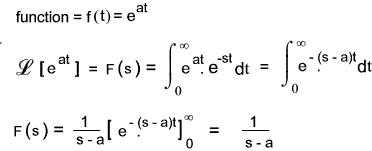From above the transform for a unit step i.e f(1) is easily obtained by setting a = 0 ( e 0.t) = 1)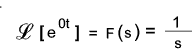From above the transform for cos ωt and sin ωt is obtained by setting a = jω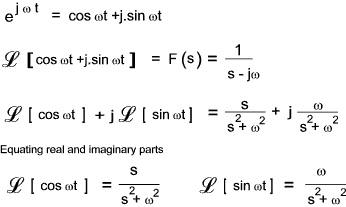Laplace transform example

An example of using Laplace transforms is provided below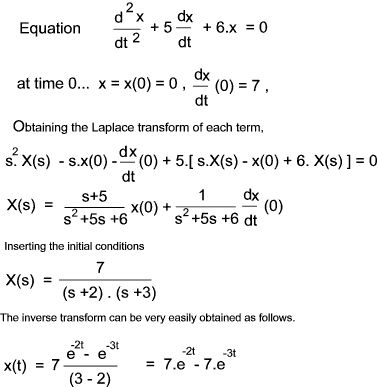The manipulation of the laplace tranform equation into a form to enable a convenient inverse transform often involves use of partial fractions...

Partial Fraction Expansion...Notes are also provided for partial fractions on webpage Partial Fractions

The splitting up of a ratio of polynomials is often necessary to produce simpler ratios from which inverse Laplace transforms are more conveniently obtained. The most favoured procedure for converting using hands-on (as opposed to using computers) is the "Heaviside cover up" procedure..

An example application including partial fraction expansion is as follows.....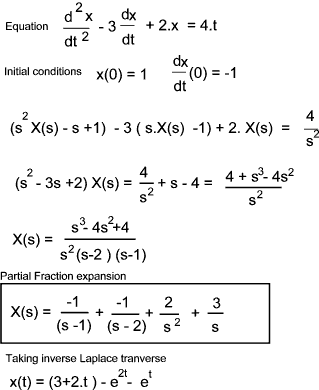Partial Fraction Expansion process using the Heaviside cover up method

The Laplace operations generally result in a ratio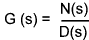This must be proper in that the order of the denominator D(s) must be higher than the numerator N(s).   If the function is not proper then the numerator N(s) must be divided by the denominator using the long division method.

The next step is to factor D(s)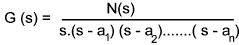a 1,a 1 etc are the roots of D(s).

D(s) is then rewritten in partial fraction form..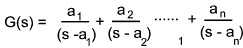To obtain a 1 simply multiply both sides of the equation by (s - a 1 ) letting s = a 1 This results in all terms on the RHS becoming zero apart from a 1...

G(s).( s-a 1 )| s = a 1 = a 1

The LHS is multiplied be (s - a 1) thus cancelling out (s - a 1)in the denominator. ... and all instances of s are then replaced by a 1
Note: If one of the terms in the numerator is s then this is simply equivalent to (s- a x) with a x = 0.

Repeated Roots....

When the denominator has repeated roots the breakdown into partial fractions is treated differently as shown below...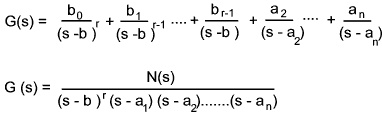The factor b 0 is obtained in exactly the same way as above..

The factor b 1 is obtained by first differentiating G(s).(s-b) with respect to ds and then substituting s with b as before. This will generally involve the differentiation d(u/v) = (vdu - udv)/v 2..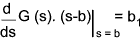The factors b 2 to b r is obtained by progressive differentiation of G(s)(s-b) and dividing the result by the factorial of the level of differentiation (if d/ds 2 then divide by 2!, if , d/ds 3 then divide by 3!(6))...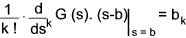Complex Roots....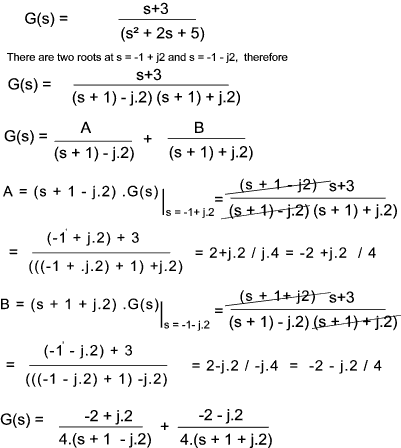Sites & Links For Control Information Vibration Data ..Table of Laplace Transforms Mathworld ..Laplace Transforms- Authoritative Information Umist ..Download .. Introduction to Laplace Transforms Partial Fraction Expansion ..Very clear explanation of principles involved Maths centre - Partial Fractions..Explanation and examples from first principles

Control Index